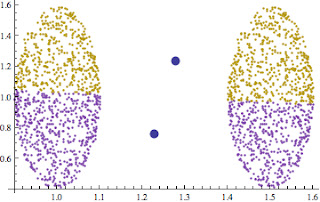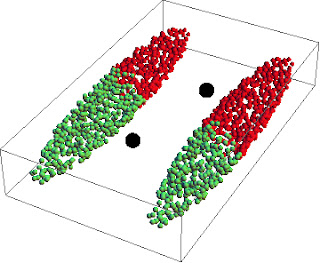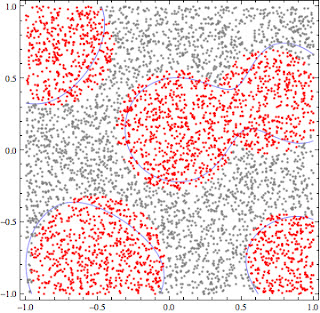Wednesday, July 13, 2011

Kmeans: an easy clustering algorithm... maybe too easy for real world problems

I was writing the first post about the document auto classification, when a friend contacted me for an opinion related to clustering algorithm.
I had a quick look at the dataset (4 components, I plotted the four combinations of 3D subsets, and I saw that the shapes were pretty much like an ellipsoid).
Let me report the call:
me:"what kind of clustering algorithm did you use?"
him: "k-means!"
me: "the stupid k-means?"
him: "yes, the data set is pretty much regular and the points are well divided so I believe kmeans should work properly...Do you think I made a mistake to implement it?"
me: "no you are a fantastic developer. More easily it cannot work with data organized like ellipsoid shape! ...try to visit wikipedia, there is a nice sample with iris data set to explain where it fails"
him: "but iris data set is a tricky set, the points are overlapped, my scenario is different: it is more easy!".
me: "ok, this evening visit my blog, I'll show you a nice sample where the data are definitely divided, but the kmeans fails!"Two set of points,  in different colors the clusters obtained, the two big points are the centroids.
Just to have another prospective, I have done the same test in 3D:Two set of points,  in different colors the clusters obtained, the two big points are the centroids.
Of course, increasing the number of centroids, the situation gets worse and worse:
I really hope that my dear friend will be convinced that doesn't exist an algorithm useful for all kind of problem!
Moreover... I would have in my job dataset "tricky" like Iris!!!
Contact me for the notebook to play with the simulations.
Stay Tuned.
PS: I'll be on vacation until the end of the month... see you soon!

Thursday, July 7, 2011

SVM classification, an intuitive explanation and some test with LIBSVM

In a previous post I focused on linear classification and on the kernel trick to make linear separable points not linear separable.
...In this post I would explain why this trick is the base to build one of the most powerful classifier: the support vectors machines (SVM).
Consider the following set of point red and blue:

As you can see, you cannot find a straight line to divide blue points from the red points.
...But as mentioned before using the kernel trick we can find a space having greater dimension where these points can be separate by  straight line.
Formally,
we are looking for a  straight line

xi ·w+b≥+1 for yi =+1 and xi ·w+b≤−1 for yi =−1
where xi represents the points (blue or red), yi =+1 to represent red points and y=−1for blue points.
and represent the unknown parameters of the straight lines.
The above equations can be combined into one set of inequalities: yi(xi ·w+b)−1≥0 ∀i
The SVM works out the classification bringing the points in a "kernel space" where the points can be divided finding a simple hyperplane.
I won't enter in technical details (the theory is based on Lagrange multipliers, kernel space, and Karush-Kuhn-Tucker Conditions) but if you are interested you can find an easy but exhaustive  description on "
I implemented in Mathematica an easy routine to call the library LIBSVM to classify the above points (contact me to obtain the notebook):
To obtain this results (overall accuracy of classification: 99,2%) i trained the SVM using a gaussian kernel.
Another 2D sample (just to highlight the hyperplane I removed the color gradations):2D pointsHyperplanes founds (in blue) for the above points

One of the interesting aspects of SVM is its vector notation: it allows a complete generalization of the problem: you can use the same algorithm to solve problem in any dimension!
For example in 3D scenario, you have:3d PointsHyperplanes found

...another example:

Now we are ready to jump into the real world and attempt to classify text documents!
In the next post I will explain a real case application of document classification using SVM.
As usual: STAY TUNED!!
cristian